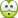# Acid dissociation constant

## Homework Statement

when 10 cm3 of a monoprotic acid C3H6O3, with concentration of 7.2g/dm3, was titrated against against 0.05M NaCl, the equivalence point reached when 15.75cm3 (ph8) of NaOH was used.
Calculate the acid dissociation constant Ka for C3H6O3.

## The Attempt at a Solution

[/B]
when it dissociate, C3H5O3- formed, but i dont know the concentration of H+ and C3H5O3- when it is in equilibrium, so how can i cal. Ka?

Quantum Defect
Homework Helper
Gold Member

## Homework Statement

when 10 cm3 of a monoprotic acid C3H6O3, with concentration of 7.2g/dm3, was titrated against against 0.05M NaCl, the equivalence point reached when 15.75cm3 (ph8) of NaOH was used.
Calculate the acid dissociation constant Ka for C3H6O3.

## The Attempt at a Solution

[/B]
when it dissociate, C3H5O3- formed, but i dont know the concentration of H+ and C3H5O3- when it is in equilibrium, so how can i cal. Ka?

You have three bits of information here with which to figure this out:

(1) 10 cm^3 of 7.2 g/dm^3 of monoprotic acid
(2) Equivalence point is reached with addition of 15.73 cm^3 of 0,05 M NaOH (typo above) ==> moles OH- = moles C3H6O3
(3) pH at equiv. point is "8" [The pH in the problem statement must refer to the pH of the equivalence point. 0.05 M NaOH does not have pH = 8 ]

So, you have at the end, a solution of the conj. base of your acid. From the pH of this solution, you can find Kb, and from this you can find Ka of the cong. acid.

epenguin
Homework Helper
Gold Member
1.

## The Attempt at a Solution

[/B]
when it dissociate, C3H5O3- formed, but i dont know the concentration of H+ and C3H5O3- when it is in equilibrium, so how can i cal. Ka?

I agree the question sounds garbled. However at pH 8 you do know the concentration of H+. The concentration of C3H6O3- is for all practical purposes equal to that of Na+ and this is an important principle that will come up in all such calculations, surely explained in your textbook, that you will need to mentally fix.If the question feels to any student weird and uncomfortable they may not be wrong as the equivalence point is a stupid place to determine a pK - the pH is changing wildly there with added base, so extremely (unnecessary!) good accuracy is being presumed here.

As this all sounded screwy I calculated (check my calcs.) using accurate atomic masses that 0.7993 mMoles acid were initially present, and 0.7875 mMoles of Na+ which is given to 4 figures, added. And is 1.5% short of equivalence. Just about makes sense.Actually that last figure tells me that the pK should be in the ballpark of a bit above 6.

And then again with the given formula there are not many options for a structure with acid group and I do not know any hydroxyacid with pK in that ballpark.Last edited:
epenguin
Homework Helper
Gold Member
I see cloveryeah has returned to the forum. He is in my black book, see my sig., unless the question here gets resolved.
Perhaps he misquoted the question, at any rate there seem to be several anomalies in it as I have laid out.
By now he must have had an answer or clarification in the form of marked homework, class discussion or something, but the thread is abandoned.
I have thought and worked more on it than a student would have if this had been a proper question. It feels like wasted time.

I've tried helping in a few too many similar timewasting unconcluded threads like this here, and with this one I finally lost patience.Last edited: